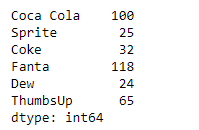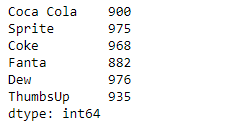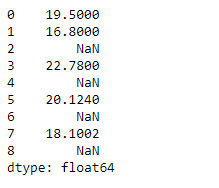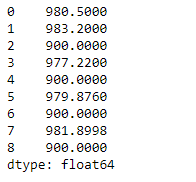# Python | Pandas Series.rsub()

Pandas series is a One-dimensional ndarray with axis labels. The labels need not be unique but must be a hashable type. The object supports both integer- and label-based indexing and provides a host of methods for performing operations involving the index.

Pandas` Series.rsub()` function return the subtraction of series and other, element-wise (binary operator rsub). It is equivalent to `other - series`, but with support to substitute a fill_value for missing data in one of the inputs.

Syntax: Series.rsub(other, level=None, fill_value=None, axis=0)

Parameter :
other : Series or scalar value
fill_value : Fill existing missing (NaN) values
level : Broadcast across a level, matching Index values on the passed MultiIndex level

Returns : result : Series

Example #1: Use `Series.rsub()` function to perform reverse subtraction of the given Series object with a scalar element-wise.

 `# importing pandas as pd ` `import` `pandas as pd ` ` `  `# Creating the Series ` `sr ``=` `pd.Series([``100``, ``25``, ``32``, ``118``, ``24``, ``65``]) ` ` `  `# Create the Index ` `index_ ``=` `[``'Coca Cola'``, ``'Sprite'``, ``'Coke'``, ``'Fanta'``, ``'Dew'``, ``'ThumbsUp'``] ` ` `  `# set the index ` `sr.index ``=` `index_ ` ` `  `# Print the series ` `print``(sr) `

Output :Now we will use `Series.rsub()` function to perform element-wise reverse subtraction of the given Series object with a scalar.

 `# perform reverse subtraction with 1000 ` `selected_items ``=` `sr.rsub(other ``=` `1000``) ` ` `  `# Print the returned Series object ` `print``(selected_items) `

Output :As we can see in the output, the `Series.rsub()` function has successfully returned the reverse subtraction of the given Series object with the scalar.

Example #2 : Use `Series.rsub()` function to perform reverse subtraction of the given Series object with a scalar element-wise. The given Series object also contains some missing values.

 `# importing pandas as pd ` `import` `pandas as pd ` ` `  `# Creating the Series ` `sr ``=` `pd.Series([``19.5``, ``16.8``, ``None``, ``22.78``, ``None``, ``20.124``, ``None``, ``18.1002``, ``None``]) ` ` `  `# Print the series ` `print``(sr) `

Output :Now we will use `Series.rsub()` function to perform element-wise reverse subtraction of the given Series object with a scalar. We will also substitute 100 at the place of all the missing values in the given Series object.

 `# perform reverse subtraction with 1000 ` `# fill 100 at the place of all missing values ` `selected_items ``=` `sr.rsub(other ``=` `1000``, fill_value ``=` `100``) ` ` `  `# Print the returned Series object ` `print``(selected_items) `

Output :As we can see in the output, the `Series.rsub()` function has successfully returned the reverse subtraction of the given Series object with the scalar.

My Personal Notes arrow_drop_upCheck out this Author's contributed articles.

If you like GeeksforGeeks and would like to contribute, you can also write an article using contribute.geeksforgeeks.org or mail your article to contribute@geeksforgeeks.org. See your article appearing on the GeeksforGeeks main page and help other Geeks.

Please Improve this article if you find anything incorrect by clicking on the "Improve Article" button below.

Article Tags :

Be the First to upvote.

Please write to us at contribute@geeksforgeeks.org to report any issue with the above content.